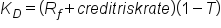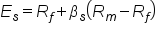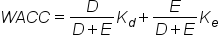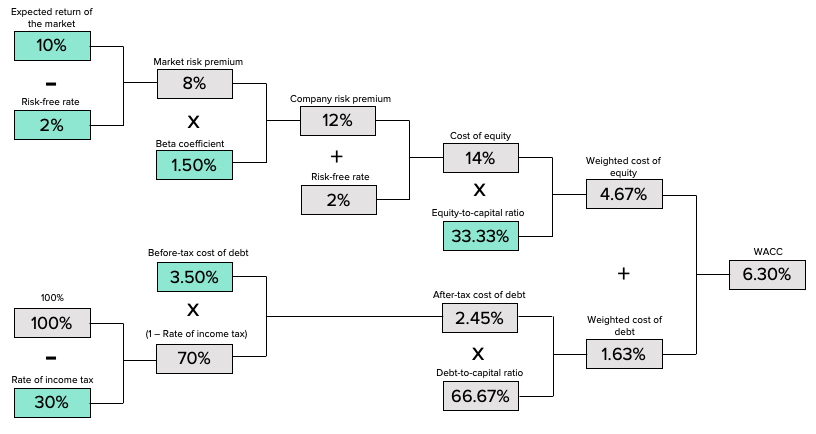# The Basics of the Cost of Capital

Author: Sophia Tutorial
##### Description:

Identify the basic concepts associated with the cost of capital.

(more)### Developing Effective Teams

*No strings attached. This college course is 100% free and is worth 1 semester credit.

37 Sophia partners guarantee credit transfer.

299 Institutions have accepted or given pre-approval for credit transfer.

* The American Council on Education's College Credit Recommendation Service (ACE Credit®) has evaluated and recommended college credit for 32 of Sophia’s online courses. Many different colleges and universities consider ACE CREDIT recommendations in determining the applicability to their course and degree programs.

Tutorial

what's covered
In this lesson, you will learn the basic concepts associated with the cost of capital. Specifically, this lesson will cover:
1. Defining the Cost of Capital
1. Net Present Value (NPV)
2. Required Rate of Return
3. Cost of Debt
4. Cost of Equity
5. Weighted Average Cost of Capital
2. Differences Between Required Return and the Cost of Capital
3. Relationship Between Financial Policy and the Cost of Capital

## 1. Defining the Cost of Capital

When defining the cost of capital, it is useful to frame it from either the borrower’s point of view (the organization) or the lender’s point of view (the investor).

• For the organization borrowing the capital, the cost of capital is the cumulative rate of interest (usually derived as an average rate, combining all capital inputs) applied to the borrowed capital to fund a project.
• From the investor’s point of view, the cost of capital is the relative required return rate, considering the risk of the investment being made.
If this all sounds a bit confusing, don’t worry. Here’s an overview of the most important concepts.

1a. Net Present Value (NPV)
The reason that capital incurs interest revolves around the simple fact that time has an impact on the valuation of capital, presenting opportunity costs and risks. To take into account the present value of future cash flows is therefore an important aspect of anticipating the rate of return on an investment. An NPV calculation will look at the forecasts for future cash flows, and discount those into present day dollars based on a given interest rate. This allows investors and organizations to determine if the cost of capital will be offset by the profits of a given investment.

1b. Required Rate of Return
From the investor’s point of view, every investment has a required rate of return for generally two reasons: the opportunity cost of foregone investments and the risk of the borrower defaulting on payments.

EXAMPLE

If an investor can get 10% return on an investment with exactly the same risk as an option with 12% return, the investor would incur an opportunity cost of 2% by investing in the 10% return option. Similarly, if two investments both yield a 10% return but present different levels of risk, an investor would make the decision based on the lowest risk option.

1c. Cost of Debt
As an organization, one of the options for sourcing capital is to pursue debt. This can either be through taking out a loan or selling corporate bonds. These options tend to have lower interest rates, and long payback periods. Debt is paid back first in the case of bankruptcy, lowering its risk as an investment, and subsequently lowering its return. The cost of this capital is calculated as:

formula

Cost of DebtIn this formula, Rf is the risk-free rate and T is the corporate rate. Tax is included in debt as debt is discounted as a deductible expense.

1d. Cost of Equity
Equity, usually represented as shares of stock, is another option for borrowing (and investing) at the organizational level. Equity’s cost is calculated via the capital asset pricing model (CAPM), as follows:

formula

Cost of EquityThe variables above are:
• Es is the expected return for a security
• Rf is the expected risk-free rate
• βs is the sensitivity to market risk
• Rm is the historical return of the market
• (Rm – Rf) is the risk premium compared to the risk-free rate
1e. Weighted Average Cost of Capital
With the above options in mind, the weighted average cost of capital (WACC) normalizes the cost of capital by combining the interest rates being incurred from both debt and equity. It can be written as:

formula

Weighted Average Cost of CapitalIn the above equation, you have:
• D as total debt
• E as total equity
• Kd as the required return on debt
• Ke as the required return on equity
If various investors require different rates of return, the equation can be altered accordingly (i.e., Kd and Ke will each be averaged based on their respective inputs). For the sake of simplicity, this WACC equation will suffice for the majority of calculations.

big idea
While each of these concepts is quite a bit more complex in implementation, this overview gives some scope as to the general definition and considerations involved in the cost of capital. Capital incurs costs due to risk, return, and the intrinsic time value of money.

term to know

Weighted Average Cost of Capital (WACC)
A calculation of the various required returns on a project, which ultimately determines the profitability required to break even.

## 2. Differences Between Required Return and the Cost of Capital

When making investments on the business level, it is critical to create a required amount of return on the project. A required return is exactly what it sounds like – the amount of profit as a percentage of the investment that will be created over a given time period.

As an investor, this required rate is a practical concept. Investors have options, and each of those options will offer a rate of return. The risk attached to those rates will fluctuate, as higher risk projects require a higher rate of return. Through establishing this required rate, the investor is stipulating their expectations on repayment of this invested capital, which the borrower will confirm and agree to repay over a set time period, usually via timed installments.

This required amount of return will ultimately equal the cost of capital, as the required rate from the investor is now a cost being put on the borrower. Now, cost of capital for a given investor will always equate to the required return. However, things get a bit more complicated when organizations fund new projects via a wide variety of stakeholders.

This is where the concept of weighted average cost of capital (WACC) enters the equation, as there may be more than one investor with varying rates of return. Intuitively, the WACC will then be a calculation which takes into account the percentage of the overall borrowed capital that each form of investment contributed, and the respective required rates.While this image goes into a bit more detail on the derivation of the cost of equity and the cost of debt, the final three boxes on the right ultimately demonstrate the way in which required rates balance out into a WACC (one for debt, one for equity). Debt tends to be a lower rate because it is paid out first if a company goes bankrupt (i.e., lower risk). Equity is a bit higher risk as it is only paid out if there is capital remaining after debts are paid, and thus equity has a higher rate (and higher risk).

Ultimately, the difference between the cost of capital and the required return is both one of perspective (borrower looks at cost of capital for a project; investors look at required return) as well as the potential for a WACC, which integrates various required returns for a single investment project.

## 3. Relationship Between Financial Policy and the Cost of Capital

Financial policy is used by companies or investors in order to determine the best way to allocate their resources. In regard to cost of capital, financial policy must be utilized in order to decide which investments have the highest return, given that resources are limited. To analyze various options, managers use valuation techniques, such as the capital asset pricing model or discounted cash flow analysis. As opposed to strictly using cost of capital, decisions must be made using opportunity cost of capital.

Opportunity cost of capital is the amount of money foregone by investing in one asset compared to another. As an investor, this can simply be a choice of one asset over another. As a company, this choice can also involve the use of current assets in new investments.

EXAMPLE

An idle piece of land could be used for a new factory; however, the opportunity cost of what else it could have been used for must be taken into consideration during analysis.

The use of financial policy in decision making does not only involve valuation. Other facets include portfolio theory, hedging, and capital structure.

Other Roles of Financial Policy Description Example
Portfolio Theory Portfolio theory is mathematical formulation of the concept of diversification in investing. It attempts to maximize the expected return of a portfolio, or a collection of investments, for a given amount of risk by carefully choosing the proportions of various assets. In other terms, portfolio theory attempts to minimize risk for a given level of expected return. To the extent prices in the stock market move differently from prices in the bond market, a collection of both types of assets can, in theory, face lower overall risk than either could individually.
Hedging Along the same lines, companies use hedging techniques to offset potential gains and losses. Hedging is the practice of taking a position in one market to offset and balance against the risk adopted by assuming a position in a contrary or opposing market or investment. Simply put, a hedge is used to reduce any substantial gains or losses suffered by an individual or an organization. Companies often use hedging techniques to offset the risk of price fluctuation for commodities, such as oil or agricultural products.
Capital Structure The capital structure of a given organization consists of the methods through which the firm has acquired, or financed its assets. This is typically done through a combination of debt, equity, or other similar types of securities. Suppose a company has sold \$20 million in equity and \$80 million in debt. This company would be 20% equity financed and 80% debt financed. A company’s ratio of debt to total financing is referred to as its leverage.

term to know

Leverage
The use of borrowed funds with a contractually determined return to increase the ability of a business to invest and earn an expected higher return (usually at high risk).
summary
In this lesson, you learned that defining the cost of capital differs for a borrower (e.g., an organization) and a lender (e.g., an investor). Calculating the net present value (NPV), the required rate of return, the cost of debt, the cost of equity, and the weighted average cost of capital (WACC) are useful when deciding how or when to raise money or invest. The difference between required return and the cost of capital is one of perspective, as the required amount of return will ultimately equal the cost of capital. There is a relationship between financial policy and the cost of capital, as companies want to make financially sound choices about where and when to allocate their resources.

Best of luck in your learning!

Source: THIS CONTENT HAS BEEN ADAPTED FROM LUMEN LEARNING'S “The Basics of the Cost of Capital” TUTORIAL.

Terms to Know
Leverage

The use of borrowed funds with a contractually determined return to increase the ability of a business to invest and earn an expected higher return (usually at high risk).

Weighted Average Cost of Capital (WACC)

A calculation of the various required returns on a project, which ultimately determines the profitability required to break even.

Formulas to Know
Cost of DebtCost of EquityWeighted Average Cost of CapitalRating